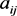﻿ 关于矩阵乘积的行列式

# 关于矩阵乘积的行列式On the Determinant of Product of Matrices

Abstract: This paper presents an expression of the determinant of the product of a matrix and its transpose. Also, an application is given.

1. 引言

$\left(n-1\right)×\left(n-1\right)$ 阶矩阵的行列式，并称 ${M}_{ij}$ 为A的对应于的余子式，称 ${A}_{ij}={\left(-1\right)}^{i+j}{M}_{ij}$${a}_{ij}$ 的代数余子式。已知A的行列式

$\mathrm{det}A=|A|=\underset{j=1}{\overset{n}{\sum }}{a}_{ij}{A}_{ij}$ .

${A}^{\text{T}}$ 表示A的转置，注意到 $|A|=|{A}^{\text{T}}|$ 。从而，

$\mathrm{det}\left(A{A}^{\text{T}}\right)=|A{A}^{\text{T}}|={|A|}^{2}$ ,

$|\begin{array}{ccc}{a}_{{i}_{1}{j}_{1}}& \cdots & {a}_{{i}_{1}{j}_{k}}\\ ⋮& & ⋮\\ {a}_{{i}_{k}{j}_{1}}& \cdots & {a}_{{i}_{k}{j}_{k}}\end{array}|$

2. 证明

$R\left(M{M}^{\text{T}}\right)

$n=m$$M=0$ ，则显然定理成立( $n=m$ 时利用两方阵的乘积的行列式等于各自的行列式的乘积)。因此下面只需讨论 $M\ne 0$$n 。首先考虑

$M=\left(\begin{array}{cccc}{a}_{11}& \cdots & {a}_{1n}& {a}_{1,n+1}\\ ⋮& & ⋮& ⋮\\ {a}_{n1}& \cdots & {a}_{nn}& {a}_{n,n+1}\end{array}\right)$ .

$\begin{array}{l}|M{M}^{\text{T}}|\\ =|\left(\begin{array}{cccc}{a}_{11}& \cdots & {a}_{1n}& {a}_{1,n+1}\\ ⋮& & ⋮& ⋮\\ {a}_{n1}& \cdots & {a}_{nn}& {a}_{n,n+1}\\ {x}_{1}& \cdots & {x}_{n}& {x}_{n+1}\end{array}\right)\left(\begin{array}{cccc}{a}_{11}& \cdots & {a}_{n1}& {x}_{1}\\ ⋮& & ⋮& ⋮\\ {a}_{1n}& \cdots & {a}_{nn}& {x}_{n}\\ {a}_{1,n+1}& \cdots & {a}_{n,n+1}& {x}_{n+1}\end{array}\right)|={|\begin{array}{cccc}{a}_{11}& \cdots & {a}_{1n}& {a}_{1,n+1}\\ ⋮& & ⋮& ⋮\\ {a}_{n1}& \cdots & {a}_{nn}& {a}_{n,n+1}\\ {x}_{1}& \cdots & {x}_{n}& {x}_{n+1}\end{array}|}^{\text{2}}\end{array}$

$A=\left(\begin{array}{cccc}{a}_{11}& \cdots & {a}_{1n}& {a}_{1,n+1}\\ ⋮& & ⋮& ⋮\\ {a}_{n1}& \cdots & {a}_{nn}& {a}_{n,n+1}\\ {x}_{1}& \cdots & {x}_{n}& {x}_{n+1}\end{array}\right)$ ，并用 ${A}_{1},{A}_{2},\cdots ,{A}_{n+1}$ 分别表示 ${x}_{1},{x}_{2},\cdots ,{x}_{n+1}$ 的代数余子式。因此，

$|M{M}^{T}|={|A|}^{2}={\left({x}_{1}{A}_{1}+\cdots +{x}_{n+1}{A}_{n+1}\right)}^{2}=|A|\underset{i=1}{\overset{n+1}{\sum }}{x}_{i}{A}_{i}$

$\begin{array}{c}|A|\left({x}_{i}{A}_{i}\right)=\left(|A|{x}_{i}\right){A}_{i}=|\begin{array}{ccccc}{a}_{11}& \cdots & {a}_{1i}{x}_{i}& \cdots & {a}_{1,n+1}\\ ⋮& & ⋮& & ⋮\\ {a}_{n1}& \cdots & {a}_{ni}{x}_{i}& \cdots & {a}_{n,n+1}\\ {x}_{1}& \cdots & {x}_{i}{}^{2}& \cdots & {x}_{n+1}\end{array}|{A}_{i}\\ =|\begin{array}{ccccc}{a}_{11}& \cdots & \underset{i=1}{\overset{n+1}{\sum }}{a}_{1i}{x}_{i}& \cdots & {a}_{1,n+1}\\ ⋮& & ⋮& & ⋮\\ {a}_{n1}& \cdots & \underset{i=1}{\overset{\text{n+1}}{\sum }}{a}_{ni}{x}_{i}& \cdots & {a}_{n,n+1}\\ {x}_{1}& \cdots & \underset{i=1}{\overset{\text{n}+1}{\sum }}{x}_{i}{}^{2}& \cdots & {x}_{n+1}\end{array}|{A}_{i}\\ =|\begin{array}{ccccc}{a}_{11}& \cdots & 0& \cdots & {a}_{1,n+1}\\ ⋮& & ⋮& & ⋮\\ {a}_{n1}& \cdots & 0& \cdots & {a}_{n,n+1}\\ {x}_{1}& \cdots & 1& \cdots & {x}_{n+1}\end{array}|{A}_{i}={A}_{i}^{2}\end{array}$

$|M{M}^{\text{T}}|=|\left(\begin{array}{ccccc}{a}_{11}& \cdots & {a}_{1n}& \cdots & {a}_{1m}\\ ⋮& & ⋮& & ⋮\\ {a}_{n1}& \cdots & {a}_{nn}& \cdots & {a}_{nm}\\ {y}_{1}& \cdots & {y}_{n}& \dots & {y}_{m}\end{array}\right)\left(\begin{array}{cccc}{a}_{11}& \cdots & {a}_{n1}& {y}_{1}\\ ⋮& & ⋮& ⋮\\ {a}_{1n}& \cdots & {a}_{nn}& {y}_{n}\\ ⋮& & ⋮& ⋮\\ {a}_{1m}& \cdots & {a}_{nm}& {y}_{m}\end{array}\right)|$

$B=\left(\begin{array}{ccccc}{a}_{11}& \cdots & {a}_{1n}& \cdots & {a}_{1m}\\ ⋮& & ⋮& & ⋮\\ {a}_{n1}& \cdots & {a}_{nn}& \cdots & {a}_{nm}\\ {y}_{1}& \cdots & {y}_{n}& \dots & {y}_{m}\end{array}\right)$ 。注意到 $m-\left(n+1\right)=k$ 。由归纳假设可知，

$|M{M}^{\text{T}}|=|B{B}^{\text{T}}|={|{B}_{1}|}^{2}+{|{B}_{2}|}^{2}+\cdots +{|{B}_{\left(\begin{array}{c}m\\ n+1\end{array}\right)}|}^{2}$ ,

${B}_{i}=\left(\begin{array}{cccc}{a}_{1{i}_{1}}& \cdots & {a}_{1{i}_{n}}& {a}_{1{i}_{n+1}}\\ ⋮& & ⋮& ⋮\\ {a}_{n{i}_{1}}& \cdots & {a}_{n{i}_{n}}& {a}_{n{i}_{n+1}}\\ {y}_{{i}_{1}}& \cdots & {y}_{n{i}_{n}}& {y}_{{i}_{n+1}}\end{array}\right),\left(1\le i\le \left(\begin{array}{c}m\\ n+1\end{array}\right)\right)$ ，且 ${y}_{{i}_{1}},{y}_{{i}_{2}},\cdots ,{y}_{{i}_{n}},{y}_{{i}_{n+1}}$ 的代数余子式分别为 ${B}_{{i}_{1}},{B}_{{i}_{2}},\cdots ,{B}_{{i}_{n}},{B}_{{i}_{n+1}}$$\left({i}_{1}<{i}_{2}<\cdots <{i}_{n+1};1\le {i}_{1},{i}_{2},\cdots ,{i}_{n+1}\le \left(\begin{array}{c}m\\ n+1\end{array}\right)\right)$ 。于是

${|{B}_{i}|}^{2}={\left({y}_{{i}_{1}}{B}_{{i}_{1}}+\cdots +{y}_{{i}_{n+1}}{B}_{{i}_{n+1}}\right)}^{2}=|{B}_{i}|\underset{r=1}{\overset{n+1}{\sum }}{y}_{{i}_{r}}{B}_{{i}_{r}}$

${j}_{1},{j}_{2},\cdots ,{j}_{r-1},{j}_{r+1},\cdots ,{j}_{n+1}$${i}_{2},\cdots ,{i}_{n+1}$

$|{B}_{j}|\left({y}_{{j}_{r}}{B}_{{j}_{r}}\right)=\left({y}_{{j}_{r}}|{B}_{j}|\right){B}_{{j}_{r}}=|\begin{array}{cccc}{y}_{{j}_{r}}{a}_{1{j}_{r}}& {a}_{1{i}_{2}}& \cdots & {a}_{1{i}_{n+1}}\\ ⋮& ⋮& & ⋮\\ {y}_{{j}_{r}}{a}_{n{j}_{r}}& {a}_{n{i}_{2}}& \cdots & {a}_{n{i}_{n+1}}\\ {y}_{{j}_{r}}{}^{2}& {y}_{{i}_{2}}& \cdots & {y}_{{i}_{n+1}}\end{array}|{B}_{{i}_{1}}$

$\begin{array}{l}|{B}_{i}|\left({y}_{{i}_{1}}{B}_{{i}_{1}}\right)+\underset{{j}_{r}\ne {i}_{1}}{\sum }|{B}_{j}|\left({y}_{{j}_{r}}{B}_{{j}_{r}}\right)\\ =|\begin{array}{cccc}{y}_{{i}_{1}}{a}_{1{i}_{1}}+\cdots +{y}_{{i}_{n+1}}{a}_{1{i}_{n+1}}& {a}_{1{i}_{2}}& \cdots & {a}_{1{i}_{n+1}}\\ ⋮& ⋮& & ⋮\\ {y}_{{i}_{1}}{a}_{n{i}_{1}}+\cdots +{y}_{{i}_{n+1}}{a}_{n{i}_{n+1}}& {a}_{n{i}_{2}}& \cdots & {a}_{n{i}_{n+1}}\\ {y}_{{i}_{1}}^{2}+\cdots +{y}_{{i}_{n+1}}^{2}& {y}_{{i}_{2}}& \cdots & {y}_{{i}_{n+1}}\end{array}|+\underset{{j}_{r}\ne {i}_{1}}{\overset{}{\sum }}|\begin{array}{cccc}{y}_{{j}_{r}}{a}_{1{j}_{r}}& {a}_{1{i}_{2}}& \cdots & {a}_{1{i}_{n+1}}\\ ⋮& ⋮& & ⋮\\ {y}_{{j}_{r}}{a}_{n{j}_{r}}& {a}_{n{i}_{2}}& \cdots & {a}_{n{i}_{n+1}}\\ {y}_{{j}_{r}}^{2}& {y}_{{i}_{2}}& \cdots & {y}_{{i}_{n+1}}\end{array}|\\ ={B}_{{i}_{1}}^{2}\end{array}$

$|M{M}^{\text{T}}|=|B{B}^{\text{T}}|={|{B}_{1}|}^{2}+{|{B}_{2}|}^{2}+\cdots +{|{B}_{\left(\begin{array}{c}m\\ n+1\end{array}\right)}|}^{2}=\frac{\underset{i=1}{\overset{\left(\begin{array}{c}m\\ n+1\end{array}\right)}{\sum }}\underset{r=1}{\overset{n+1}{\sum }}{B}_{{i}_{r}}^{2}}{m-n}$

3. 推广与应用

$|{M}_{1}{M}_{2}|=\underset{1\le {k}_{1}<{k}_{2}<\cdots <{k}_{n}\le m}{\sum }{M}_{1}\left(\begin{array}{cccc}1& 2& \cdots & n\\ {k}_{1}& {k}_{2}& \cdots & {k}_{n}\end{array}\right){M}_{2}\left(\begin{array}{cccc}{k}_{1}& {k}_{2}& \cdots & {k}_{n}\\ 1& 2& \cdots & n\end{array}\right)$ .

$M\left(\begin{array}{cccc}{i}_{1}& {i}_{2}& \cdots & {i}_{r}\\ {j}_{1}& {j}_{2}& \cdots & {j}_{r}\end{array}\right)$

NOTES

*通讯作者。

 Lewis, D.W. (1991) Matrix Theory. World Scientific, Singapore.
https://doi.org/10.1142/1424

 Rotman, J.J. (2000) A First Course in Abstract Algebra. Prentice Hall, Upper Saddle River.

 李尚志. 线性代数[M]. 北京: 高等教育出版社, 2006: 213-214.

 北京大学数学系前代数小组. 高等代数[M]. 北京: 高等教育出版社, 2013: 175-180.

Top